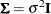### Example 27.4 Testing Covariance Patterns

In the test for sphericity, a covariance matrix is hypothesized to be a constant multiple of an identity matrix. That is, the null hypothesis for the population covariance matrix iswhereis an unknown positive constant andis an identity matrix. When this covariance pattern is applied to the sales data in Example 27.1, this hypothesis states that all four variables have the same varianceand are uncorrelated with each other. This model is more restricted than the uncorrelatedness model in Example 27.3, which requires uncorrelatedness but does not require equal variances. Use the following specification to conduct a sphericity test for the sales data:

proc calis data=sales;
mstruct var=q1-q4;
matrix _cov_ [1,1] = 4*sigma_sq;
run;


This specification is similar to that of Example 27.3. The major difference is the MATRIX statement specification. The current example uses a parameter name sigma_sq to represent the unknown variance parameter, whereas Example 27.3 specifies only the locations of the four free variance parameters.

The current MATRIX statement specification uses a shorthand notation. On the left-hand side of the equal sign, [1,1] indicates the starting location of the covariance matrix. The matrix entries automatically proceed to [2,2], [3,3] and so on, depending on the length of the parameter list specified on the right-hand sign of the equal sign. For example, if there is just one parameter on the right-hand side, the matrix specification contains only [1,1]. In the current example, the specification 4*sigma_sq means that sigma_sq appears four times in the specification. As a result, the preceding MATRIX statement specification is equivalent to the following statement:

matrix _cov_ [1,1] = sigma_sq,
[2,2] = sigma_sq,
[3,3] = sigma_sq,
[4,4] = sigma_sq;


This matrix is what is required by the sphericity test. Use either the expanded notation or the shorthand notation for specifying the covariance pattern. For details about various types of shorthand notation for parameter specifications, see the MATRIX statement.

Output 27.4.1 shows the initial model specification under the test of sphericity. All the diagonal elements are labeled with the same name sigma_sq, indicating that they are the same parameter.

Output 27.4.1: Covariance Model under Sphericity for the Sales Data

Initial MSTRUCT _COV_ Matrix
q1 q2 q3 q4
q1
 . [sigma_sq]
 0
 0
 0
q2
 0
 . [sigma_sq]
 0
 0
q3
 0
 0
 . [sigma_sq]
 0
q4
 0
 0
 0
 . [sigma_sq]

Output 27.4.2 shows that the model fit chi-square is 31.5951 (df = 9, p = 0.0002). This means that the covariance pattern under the sphericity hypothesis is not supported.

Output 27.4.2: Fit Summary of the Sphericity Test for the Sales Data

Fit Summary
Chi-Square 31.5951
Chi-Square DF 9
Pr > Chi-Square 0.0002

Output 27.4.3 shows the estimated covariance matrix under the sphericity hypothesis. The variance estimate for all four diagonal elements is 0.9587 (standard error=0.1880).

Output 27.4.3: Fitted Covariance Matrix under the Sphericity Hypothesis for the Sales Data

MSTRUCT _COV_ Matrix: Estimate/StdErr/t-value
q1 q2 q3 q4
q1
 0.9587 0.1880 5.0990 [sigma_sq]
 0
 0
 0
q2
 0
 0.9587 0.1880 5.0990 [sigma_sq]
 0
 0
q3
 0
 0
 0.9587 0.1880 5.0990 [sigma_sq]
 0
q4
 0
 0
 0
 0.9587 0.1880 5.0990 [sigma_sq]

This example shows how you can specify a simple covariance pattern by using the MATRIX statement. Use the same parameter names to constrain variance parameters that are supposed to be the same under the model. Constraining parameters by using the same parameter names is applicable not only to the MSTRUCT models, but also to more complicated covariance structure models, such as multiple-group modeling (see Example 27.19 and Example 27.21).

The MSTRUCT modeling language is handy when you can directly specify the covariance pattern or structures in your model. However, in most applications of structural equation modeling, it is difficult to specify such direct covariance structures. Instead, the covariance structures are usually implied from the functional relationships among the variables in the model. Using the MSTRUCT modeling language in such a situation is not easy. Fortunately, PROC CALIS supports other modeling languages that enable you to specify the functional relationships among variables. The functional relationships can be in the form of a set of path-like descriptions, a system of linear equations, or parameter specifications in matrices. See Example 27.6 for an introduction to using the PATH modeling language for specifying path models.